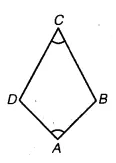# Draw a rough figure of a quadrilateral that is not a parallelogram but has exactly two opposite

Draw a rough figure of a quadrilateral that is not a parallelogram but has exactly two opposite angles of equal measure.A rough figure of a quadrilateral ABCD that is not a parallelogram has been drawn with exactly two opposite angles of equal measure such that ∠A – ∠C which is a kite as an example.